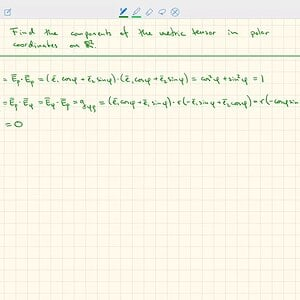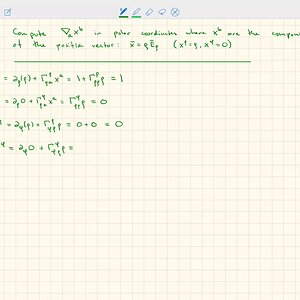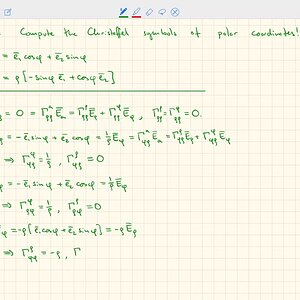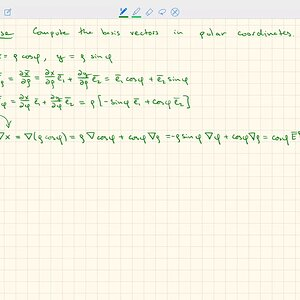# Polar coordinates Definition and 64 Discussions

In mathematics, the polar coordinate system is a two-dimensional coordinate system in which each point on a plane is determined by a distance from a reference point and an angle from a reference direction. The reference point (analogous to the origin of a Cartesian coordinate system) is called the pole, and the ray from the pole in the reference direction is the polar axis. The distance from the pole is called the radial coordinate, radial distance or simply radius, and the angle is called the angular coordinate, polar angle, or azimuth. The radial coordinate is often denoted by r or ρ, and the angular coordinate by φ, θ, or t. Angles in polar notation are generally expressed in either degrees or radians (2π rad being equal to 360°).
Grégoire de Saint-Vincent and Bonaventura Cavalieri independently introduced the concepts in the mid-17th century, though the actual term polar coordinates has been attributed to Gregorio Fontana in the 18th century. The initial motivation for the introduction of the polar system was the study of circular and orbital motion.
Polar coordinates are most appropriate in any context where the phenomenon being considered is inherently tied to direction and length from a center point in a plane, such as spirals. Planar physical systems with bodies moving around a central point, or phenomena originating from a central point, are often simpler and more intuitive to model using polar coordinates.
The polar coordinate system is extended to three dimensions in two ways: the cylindrical and spherical coordinate systems.

View More On Wikipedia.org
1. ### Dipole in polar coordinates

I don't know how to get the result referring to the previous task. Is my decision correct?
2. ### A Converting this vector into polar form

In the following%3A%20https://pubs.rsc.org/en/content/articlehtml/2013/sm/c3sm00140g?casa_token=3O_jwMdswQQAAAAA%3AaSRtvg3XUHSnUwFKEDo01etmudxmMm8lcU4dIUSkJ52Hzitv2c_RSQJYsoHE1Bm2ubZ3sdt6mq5S-w'] paper, the surface velocity for a moving, spherical particle is given as (eq 1)...
3. ### A Polar Fourier transform of derivatives

The 2D Fourier transform is given by: \hat{f}(k,l)=\int_{\mathbb{R}^{2}}f(x,y)e^{-ikx-ily}dxdy In terms of polar co-ordinates: \hat{f}(\rho,\phi)=\int_{0}^{\infty}\int_{-\pi}^{\pi}rf(r,\theta)e^{-ir\rho\cos(\theta-\phi)}drd\theta For Fourier transforms in cartesian co-ordinates, relating the...
4. ### Integration of acceleration in polar coordinates

I made this exercise up to acquire more skill with polar coordinates. The idea is you're given the acceleration vector and have to find the position vector corresponding to it, working in reverse of the image. My attempts are the following, I proceed using 3 "independent" methods just as you...
5.### SH2372 General Relativity (5X): Metric components in polar coordinates

6.### SH2372 General Relativity (4X): Covariant derivative of the position vector in polar coordinates

7.### SH2372 General Relativity (3X): Christoffel symbols in polar coordinates

8.9. ### I Moving center of coordinates in the polar graph

I have a function in polar coordinates: t (rho, phi) = H^2 / (H^2 + rho^2) (1) I have moved the center to the right and want to get the new formulae. I use cartesian coordinates to simplify the transformation (L =...
10. ### A Analytical solution for an integral in polar coordinates?

Hi, I am trying to find open-form solutions to the integrals attached below. Lambda and Eta are positive, known constants, smaller than 10 (if it helps). I would appreciate any help! Thank you!
11. ### Differential position vector

I had an equation. $$T=\frac{1}{2}m[\dot{x}^2+(r\dot{\theta})^2]$$ Then, they wrote that $$\mathrm dr=\hat r \mathrm dr + r \hat \theta \mathrm d \theta + \hat k \mathrm dz$$ I was thinking how they had derived it. The equation is looking like, they had differentiate "something". Is it just an...
12. ### Simmons 7.11 Family of curves of a differential equation that intersect at angle pi/4

>10. Let a family of curves be integral curves of a differential equation ##y^{\prime}=f(x, y) .## Let a second family have the property that at each point ##P=(x, y)## the angle from the curve of the first family through ##P## to the curve of the second family through ##P## is ##\alpha .## Show...

45. ### Solving for the trajectory in the polar coordinate system

Homework Statement On the surface of a river at ##t=0## there is a boat 1 (point ##F_0##) at a distance ##r_0## from the point ##O## (the coordinate beginning) which is on the right side of the coast (picture uploaded below). A line ##OF_0## makes an angle ##θ_0=10°## with the ##x-axis## whose...
46. ### Having a hard time writing equations of motion....

Homework Statement [/B] Homework Equations The Attempt at a Solution [/B]
47. ### Equations of Motion of a Mass Attached to Rotating Spring

1. Homework Statement A particle of mass m is attached to the end of a light spring of equilibrium length a, whose other end is fixed, so that the spring is free to rotate in a horizontal plane. The tension in the spring is k times its extension. Initially the system is at rest and the...
48. ### Acceleration of the end of a hinged rod in a pulley system

Homework Statement As shown in image. 2. Homework Equations Moment of inertia of pulley = 1/2*M*R^2 Moment of inertia of rod (about end) = 1/3*M*L^2 Acceleration of end of rod in theta direction = L*α Acceleration of end of rod in radial direction = L*ω^2 The Attempt at a Solution...
49. ### Polar coordinates of a vector

Note: All bold and underlined variables in this post are base vectors I was reading the book 'Introduction To Mechanics' by Kleppner and Kolenkow and came across an example I don't quite understand. The example is this: a bead is moving along the spoke of a wheel at constant speed u m/s. The...
50. ### Polar Coordinates

1. The question The position of a particle is given by r(t) = acos(wt) i + bsin(wt) j. Assume a and b are both positive and a > b. The plane polar coordinates of a particle at a time t equal to 1/8 of the time period T will be given by _ Homework Equations r(t) = acos(wt) i + bsin(wt) j. The...